# Fractional Position on a Number Line Online Quiz

Following quiz provides Multiple Choice Questions (MCQs) related to Fractional Position on a Number Line. You will have to read all the given answers and click over the correct answer. If you are not sure about the answer then you can check the answer using Show Answer button. You can use Next Quiz button to check new set of questions in the quiz.Q 1 - What is the position of A on the number line? Write your answer as a fraction.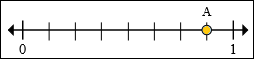A - 7/6

B - 6/8

C - 7/8

D - 5/8

### Explanation

Step 1:

The number of parts between 0 and 1 are 8.

Step 2:

We find that A is on the 7th mark to the right of 0.

So A is at 7/8 mark on the number line.

Q 2 - What is the position of B on the number line? Write your answer as a fraction.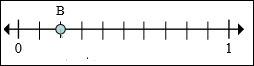A - 2/8

B - 2/10

C - 3/10

D - 4/10

### Explanation

Step 1:

The number of parts between 0 and 1 are 10.

Step 2:

We find that B is on the 2nd mark to the right of 0.

So B is at 2/10 mark on the number line.

Q 3 - What is the position of B on the number line? Write your answer as a fraction.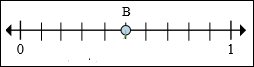A - 2/10

B - 3/10

C - 5/8

D - 5/10

### Explanation

Step 1:

The number of parts between 0 and 1 are 10.

Step 2:

We find that B is on the 5th mark to the right of 0.

So B is at 5/10 mark on the number line.

Q 4 - What is the position of A on the number line? Write your answer as a fraction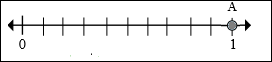A - 10/10

B - 9/10

C - 8/10

D - 7/10

### Explanation

Step 1:

The number of parts between 0 and 1 are 10.

Step 2:

We find that A is on the 10th mark to the right of 0.

So A is at 10/10 or 1 mark on the number line.

Q 5 - What is the position of C on the number line? Write your answer as a fraction.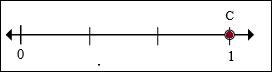A - 1/3

B - 3/3

C - 2/3

D - 4/3

### Explanation

Step 1:

The number of parts between 0 and 1 are 3.

Step 2:

We find that C is on the 3rd mark to the right of 0.

So C is at 3/3 or 1 mark on the number line.

Q 6 - What is the position of A on the number line? Write your answer as a fraction.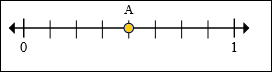A - 3/8

B - 6/8

C - 5/8

D - 4/8

### Explanation

Step 1:

The number of parts between 0 and 1 are 8.

Step 2:

We find that A is on the 4th mark to the right of 0.

So A is at 4/8 mark on the number line.

Q 7 - What is the position of C on the number line? Write your answer as a fraction.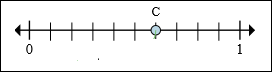A - 6/10

B - 5/10

C - 7/10

D - 6/8

### Explanation

Step 1:

The number of parts between 0 and 1 are 10.

Step 2:

We find that C is on the 6th mark to the right of 0.

So C is at 6/10 mark on the number line.

Q 8 - What is the position of A on the number line? Write your answer as a fraction.A - 2/6

B - 3/6

C - 4/6

D - 5/6

### Explanation

Step 1:

The number of parts between 0 and 1 are 6.

Step 2:

We find that A is on the 4th mark to the right of 0.

So A is at 4/6 mark on the number line.

Q 9 - What is the position of A on the number line? Write your answer as a fraction.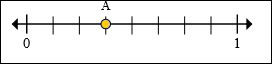A - 5/8

B - 3/10

C - 4/8

D - 3/8

### Explanation

Step 1:

The number of parts between 0 and 1 are 8.

Step 2:

We find that A is on the 3th mark to the right of 0.

So A is at 3/8 mark on the number line.

Q 10 - What is the position of C on the number line? Write your answer as a fraction.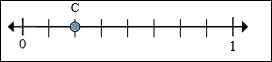A - 2/8

B - 3/8

C - 4/8

D - 2/10

### Explanation

Step 1:

The number of parts between 0 and 1 are 8.

Step 2:

We find that A is on the 2nd mark to the right of 0.

So A is at 2/8 mark on the number line.

fractional_position_on_number_line.htm